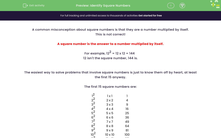# Identify Square Numbers

In this worksheet, students find square numbers and apply knowledge of square numbers to solve problems.Key stage:  KS 4

Year:  GCSE

GCSE Subjects:   Maths

GCSE Boards:   Pearson Edexcel, OCR, Eduqas, AQA,

Curriculum topic:   Number, Indices and Surds

Curriculum subtopic:   Structure and Calculation Powers and Roots

Popular topics:   Square Numbers worksheets

Difficulty level:#### Worksheet Overview

A common misconception about square numbers is that they are a number multiplied by itself.

This is not correct!

A square number is the answer to a number multiplied by itself.

For example, 122 = 12 x 12 = 144

12 isn't the square number, 144 is.

The easiest way to solve problems that involve square numbers is just to know them off by heart, at least the first 15 anyway.

The first 15 square numbers are:

 12 1 x 1 1 22 2 x 2 4 32 3 x 3 9 42 4 x 4 16 52 5 x 5 25 62 6 x 6 36 72 7 x 7 49 82 8 x 8 64 92 9 x 9 81 102 10 x 10 100 112 11 x 11 121 122 12 x 12 144 132 13 x 13 169 142 14 x 14 196 152 15 x 15 225

Let's practise using these numbers in calculations now.

Squaring an integer:

e.g. Find the value of 62

6 x 6 = 36

Squaring a decimal:

One thing examiners like to do is to tie in one topic with another.

If you ever see a question involving squaring a decimal without a calculator, it will be based around the square numbers which you would already have knowledge of.

e.g. Find the value of 1.22

This just means we need to find the value of: 1.2 x 1.2

If we look at our table, we can see that:

12 x 12 = 144

So 1.2 x 1.2 = 1.44 when we put out decimal point back in

Great focus!

In this activity, you will find square numbers and apply your knowledge of square numbers to solve problems.

Now let's attack some questions and really consolidate our knowledge.

### What is EdPlace?

We're your National Curriculum aligned online education content provider helping each child succeed in English, maths and science from year 1 to GCSE. With an EdPlace account you’ll be able to track and measure progress, helping each child achieve their best. We build confidence and attainment by personalising each child’s learning at a level that suits them.

Get started